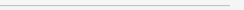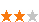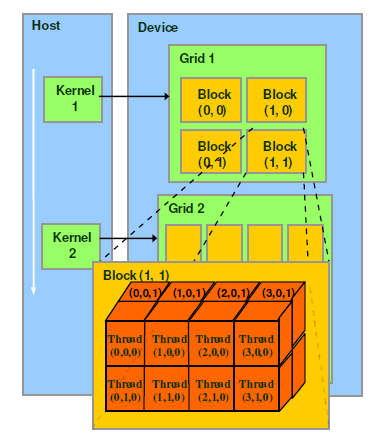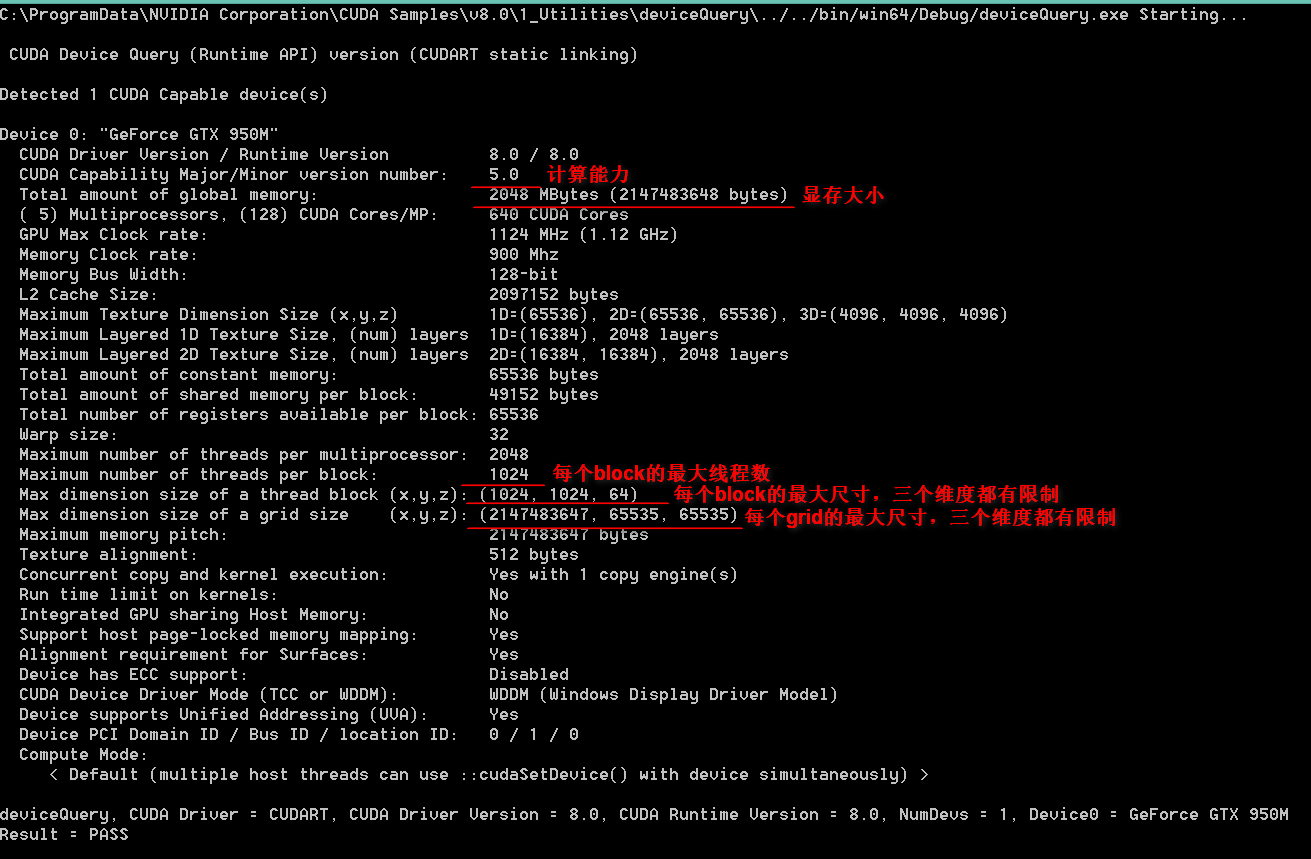您可以捐助，支持我们的公益事业。 1元 10元 50元 认证码：必填求知 文章 文库 Lib 视频 iProcess 课程 角色 咨询 工具 讲座 Modeler Code要资料订阅捐助833 次浏览     评价： 好 中 差
2018-8-24

 编辑推荐: 本文来自于cnblogs，文章主要对CUDA方案下的高并行的计算的详细阐述，同时对其他几种方案进行了描述。

1. 计算规模初体验

 0 2 0 2 0 0 0 0 0 0 0 40 0 0 35 0 0 53 0 44 0 0 0 0 0 0 0 0 0 0 0 0 1 148 0 0 0 0 0 0 0 0 0 0 1133 0 1 0 0 1820 0 0 0 2 0 0 0 1 0 0 0 0 0 33 1 0 0 0 0 0 0 0 0 0 231 0 0 0 0 402 0 0 0 0 0 0 0 0 0 0 0 0 6 0 0 0 0 0 0 0 0 0 0 0 1 0 0 0 0 0 1 0 6 0 0 0 0 0 0 0 0 0 0 0 0 0 0 0 5 0 0 0 0 0 0 0 0 0 0 0 0 0 0 0 0 0 0 0 0 0 ... ... ... ...

2. MATLAB多线程

MATLAB写起来最简单，计算相关系数和P值都不用操心，一行自带的函数调用就完成。打开MATLAB左下角的并行池，MATLAB将会自动寻找到机子上有的物理核心，并分配与物理核心数相同的worker。比如我的电脑是4核8线程，它只能开4个worker，不识别虚拟核心。

 t1 = clock; disp('>> loading ...'); A = importdata('D:/MASTER2016/5.CUDA/data-ID-top30-kv3.txt'); b = A'; %由于MATLAB只计算列与列之间的相关系数，因此需要转置操作 disp(etime(clock,t1)); num = size(b, 2); disp('>> calculating ...'); fid = fopen('D:/MASTER2016/5.CUDA/result-matlab.txt', 'wt'); for i = 1 : num for j = i+1 : num [m, n] = corr(b(:, i), b(:, j), 'type', 'Spearman', 'tail', 'both'); if isnan(n) || n>0.05 continue; end fprintf(fid, 'X%d\tX%d\t%d\t%d\n', i, j, m, n); end end fclose(fid); disp('>> OK!');

3. Python多线程

Python语言由于本身的体质问题，Cython下不能调用多核，只能用多线程。理论上是这样，但还是有很多扩展包能够充分压榨出多核CPU性能，例如multiprocessing是其中的佼佼者。multiprocessing用起来也非常简单，考虑到CPU的多核运算下，每颗核心的算力还是很可观的，所有不能把每个计算组都拆成并行线程，那样内存的读写开销反而会使CPU一直在等待状态，不能一直满负载工作。鉴于此，我设计9879895组线程，每组代表某个特定行与剩下的各个数据行形成的数据组。这样每组线程下的运算量还是比较大的，能使CPU尽可能全在满负载状态。

 # coding=utf-8 import math import multiprocessing import time import scipy.stats as stats def calculate2(i, X, all_glb, data_array_glb): all = all_glb.value result = [] for j in range(i + 1, all): x = X y = data_array_glb[j] if math.fsum(x) == 0 or math.fsum(y) == 0: continue corr, p = stats.spearmanr(x, y) if p > 0.05: continue result.append([i + 1, j + 1, corr, p]) return result if __name__ == "__main__": multiprocessing.freeze_support() input_file = 'D:/MASTER2016/5.CUDA/data-ID-top30-kv3.txt' output_file = 'D:/MASTER2016/5.CUDA/result-python.txt' print '>> loading ...' start = time.clock() data = open(input_file) data_array = [] for line in data: data_array.append(map(int, line.strip().split(' '))) data.close() print time.clock()-start, 's' print '>> calculating ...' results = [] pool_size = 8 pool = multiprocessing.Pool(processes=pool_size) all = len(data_array) manager = multiprocessing.Manager() all_share = manager.Value('i', int(all)) data_array_share = manager.list(data_array) for i in range(all): data_X = data_array[i] results.append(pool.apply_async(calculate2, args=(i, data_X, all_share, data_array_share))) pool.close() pool.join() print time.clock() - start, 's' data_array = None print '>> saving ...' data2 = open(output_file, 'w') for res in results: temp_list = res.get() for temp in temp_list: data2.write('X'+str(temp)+'\t'+'X'+str(temp) +'\t'+str(temp)+'\t'+str(temp)+'\n') print time.clock()-start, 's' data2.close()

4. Spark方案

Spark方案我并没有写完，因为写着写着就感觉到。。。肯定还是不行，CPU的算力也就那样了。就算调12台机器一起跑，也不适合用CPU下的线程模型解决问题了。

5. CUDA方案

CUDA方案下，首先必须清晰地设计好线程模型，即：我需要用到几块GPU？我需要在每块GPU上设计多少个block？每个block设计多少个线程？每个线程分配多少运算量？这四个问题基本决定了CUDA程序的性能和复杂度。Talk is cheap. Show me the code:

 #include #include #include #include #include #include "cuda_runtime.h" #include "device_launch_parameters.h" // 定义总数据矩阵的行数和列数 #define ROWS 15000 #define COLS 30// 定义每一块内的线程个数，GT720最多是1024（必须大于总矩阵的列数：30） #define NUM_THREADS 1024 bool InitCUDA() { int count; cudaGetDeviceCount(&count); if (count == 0) { fprintf(stderr, "There is no device.\n"); return false; } int i; for (i = 0; i < count; i++) { cudaDeviceProp prop; if (cudaGetDeviceProperties(&prop, i) == cudaSuccess) { if (prop.major >= 1) { break; } } } if (i == count) { fprintf(stderr, "There is no device supporting CUDA 1.x.\n"); return false; } cudaSetDevice(i); return true; }__device__ float meanForRankCUDA(int num) { float sum = 0; for (int i = 0; i <= num; i++) { sum += i; } return sum / (num + 1); } __device__ float meanForArrayCUDA(float array[], int len) { float sum = 0; for (int i = 0; i < len; i++) { sum += array[i]; } return sum / len; } __device__ float spearmanKernel(int Xarray[], int Yarray[]) { //1，对原先的数据进行排序，相同的值取平均值